2018-07-31 12:07:14

# 排列组合

## -“嗯，可以的，我们来试试。”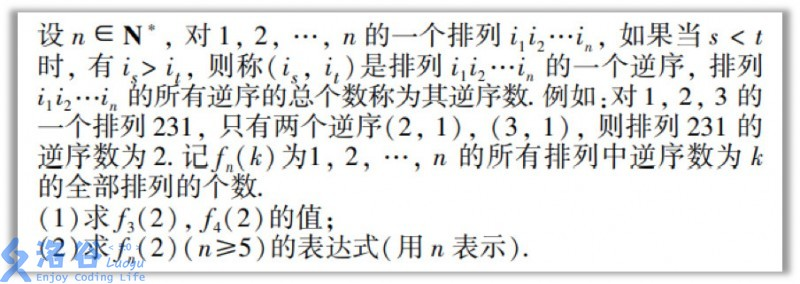## 排列

### ●普通排列

123 132 213 231 312 321

123 124 132 134 142 143 213 214 231 234 241 243

312 314 321 324 341 342 412 413 421 423 431 432

$$P_n^m$$

$$P_n^m=n*(n-1)*(n-2)*...*(n-m+1)$$

$$P_n^m=\frac{n!}{(n-m)!}$$

$$(0!)=1$$ $$P_n^0=1$$

#### ●圆排列

$$ans=\frac{P_7^4}{4}=\frac{7!}{(7-4)!*4}=210$$

$$ans=\frac{n!}{(n-m)!*m}$$

### ●带有重复元素的排列

$$cnt_{1}*cnt_{2}*...*cnt_{m}$$

$$\frac{n!}{cnt_{1}*cnt_{2}*...*cnt_{m}}$$

## 组合

### ●普通组合

$$C_n^m=\frac{P_n^m}{m!}=\frac{n!}{(n-m)!*m!}$$

### ●杨辉三角与组合数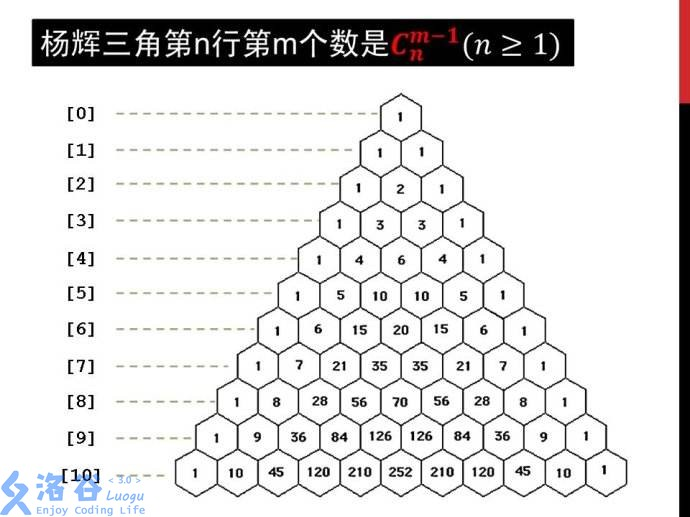（1）不含第n个数，方案数为C(n-1,m)。

（2）含第n个数，方案数为C(n-1,m-1)。

$$C_n^m=C_{n-1}^m+C_{n-1}^{m-1}$$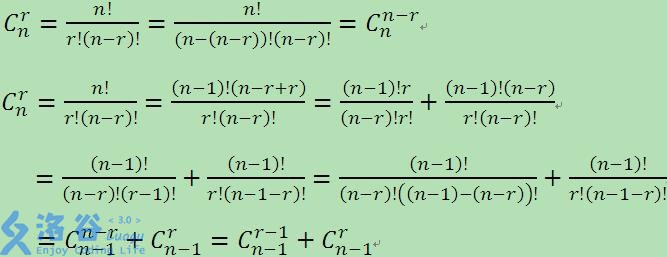### ●二项式定理

$$(a+b)^n=\sum_{m=0}^nC_n^ma^{n-m}b^m$$

$$(a+b)^n=(a+b)(a+b)...(a+b)$$

### ●卡特兰数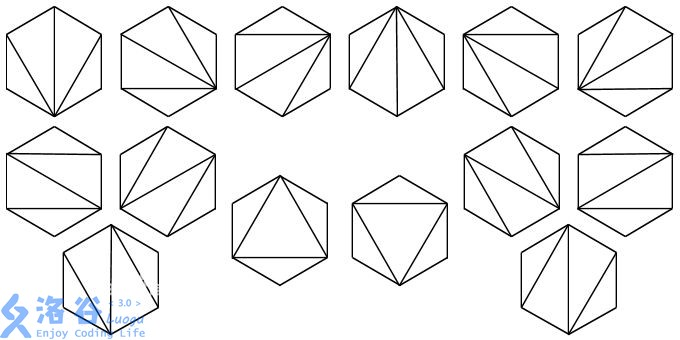$$Cat(0)=1,Cat(1)=1$$ $$Cat(n)=\sum_{i=0}^{n-1}Cat(i)*Cat(n-1-i)$$ $$=\frac{C_{2n}^n}{n+1}$$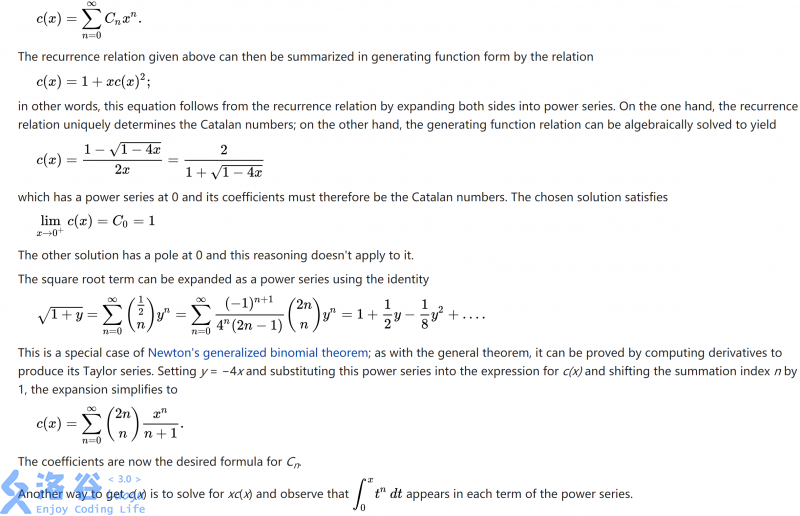1.如图所示的路径数量问题（从左下角走到右上角，只能在红线的右侧走）。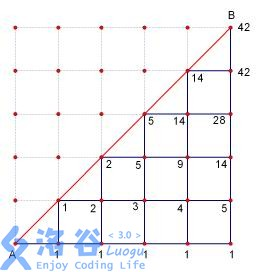2.出栈方案问题（luogo P1044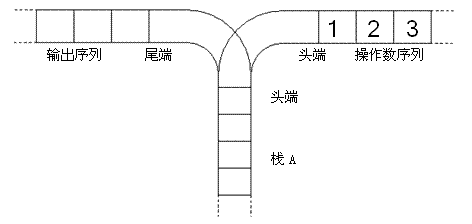van♂结撒花★,°:.☆(￣▽￣)/ $:*.°★* 。 -“喂(#`O′)，等等，江苏的那道高考题该怎么写啊？” ###●那道题的解法 由于题目在文章的开头，所以这里再发一遍： ![题目](https://cdn.luogu.com.cn/upload/pic/26061.png) 对不起，发错了。这才是真正的题目： ![真正的题目](https://cdn.luogu.com.cn/upload/pic/26014.png) 这题的的第一问过水，我们直接看第二问。 我们在排好序的原序列上有两种操作可以产生一个逆序对为2的排列： （1）任意交换两对相邻的数，共有C(n-1,2)种方案。 （2）将一个数向前移动两次，共有(n-2)种方案。 $$fn(2)(n\ge5)=C{n-1}^2+(n-2)=\frac{(n-1)(n-2)}{2}+n-2=\frac{n^2-n-2}{2}$$ ##真正的完结撒花！*★,°*:.☆(￣▽￣)/$ :.°★

• star
首页